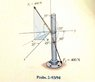# Dot products and angle between cables

musicmar

## Homework Statement

This is actually for an engineering class, but it's pretty basic vector addition.

Determine the angle θ between the two cables attached to the post.

I've attached the picture.

## The Attempt at a Solution

From the picture:
F1:
α=?
β=?
γ= 35°

F2:
α= 45°
β= 60°
γ= 120°

First, to find F2, I used the angles and the formula cos(α)=(F2x/F2)

F2=<282.84,200,-200>

To find F1, I used the 35° from the vertical to find the z component, then used the 20° in the xy plane to find the x and y components.

F1=<215.59,-78.47,327.66>

F1·F2=F1F2cosθ

θ=cos-1(F1·F2/4002)
=cos-1(-600.84/4002)=90.2°

According to the back of the book, the answer is 97.3°. So, I've made a mistake somewhere. Any help finding it would be greatly appreciated.

#### Attachments

•engr220_2-94.jpg
27.6 KB · Views: 984